# Working With Time- Node-Red Programming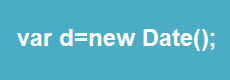Working With Time is very common in all programming languages.

In this tutorial I want to take you through the basics of dealing with dates and times in JavaScript and some code snippets that I commonly use in my flows.

The key to working with time in JavaScript is the Date object.

So the first thing you need to do is to get a date object. There are several ways of doing this but the most basic is:

`var d=new Date();`

This creates a date object with the current date and time if you print it it looks like this.

```2020-06-08T16:14:36.457Z
```

However this is not the only way you can create a new Date object there are several other methods which you might find useful:

• new Date()
• new Date(year, month, day, hours, minutes, seconds, milliseconds)
• new Date(milliseconds)
• new Date(date string)

Example:. Creating a Object Using a Date String

`var date = new Date('September 22, 2018 13:22:13')`

### JavaScript Time Stamp and Unix Time stamp

JavaScript stores time in milliseconds since Jan 1st 1970 (UTC) (Unix Time).

However if you have data with a Unix time stamp then this will be seconds and not milliseconds.

So to convert a Unix time stamp into a JavaScript time stamp you need to multiple by 1000.

When working with time the most common thing to do is to get a Unix type time stamp using the getTime method as shown in the screen shot below: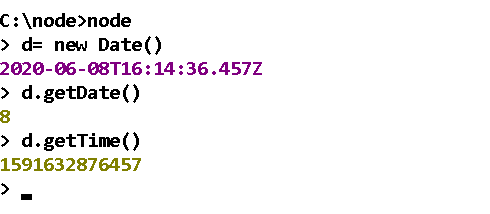If you have an existing Unix type timestamp then you can also use this timestamp to create the date object from that time stamp.:

`var d=new Date(timestamp);`

This is common when you are ready to display data and need to convert the time stamp into a more user friendly form.

Generally we use times in Unix time stamp format which makes them very easy to work with , and convert them into a human readable format to displaying them.

Examples:

1.Calculating interval between events

```var d=new Date();
var start_time=d.getTime()
//something happens here
d=new Date();
var end_time=d.getTime()
var difference =end_time-start_time;
```

2. Comparing Times

```var d=new Date();
var time1=d.getTime()
d=new Date();
time2=d.getTime()
if(time1<=time2)
//do something
```

### Converting Dates for Display

The following table shows the common Date display forms

 JavaScript Date Formats ISO Date “2015-03-25” =year-month-day (The International Standard) Short Date “03/25/2015” =month/day/year Long Date “Mar 25 2015” or “25 Mar 2015”

You can extract the current day, month,year and seconds etc from the Date object using the get methods which all return local time:

```var d=new Date();
var day=d.getDate()  //day as a number 0 -31
var weekday=d.getDay()  //-weekday as a number  0-6
var month=d.getMonth() // month as a number 0-11
var year=d.getFullYear() //year as a four digit number (yyyy)
var hours=d.getHours() //the hour (0-23)
var minutes=d.getMinutes() //-the minute (0-59)
var seconds=d.getSeconds() //-the seconds (0-59)
```

However there is also a UTC equivalent of each that returns the time in UTC e.g

var hours=d.getUTCHours() //the hour (0-23)

The screen shot below show it’s use: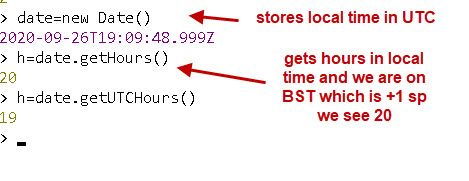To get the month by name use:

```var months={1:"January",2:"February",3:"March",4:"April",5:"May",6:"June",7:"July",8:"August",9:"September",10:"October",11:"November",12:"December"};

var month=d.getMonth();

var month_name=months[month];
```

You can do a similar thing for weekdays

### Formatting Time for Display

When displaying dates and time on graphs,tables etc we usually want it to be in human readable form.

In addition we usually want the display a month as 01 for Janauary and not just 1. The same also applied for days

Example 1- Create date of form day/month/year e.g.09/04/2020.

This example uses the get methods

```var d=new Date();
var day=d.getDate();
var month=d.getMonth();
var year=d.getFullYear();
if(day<=9)
day="0"+day;
if(month<=9)
month="0"+month;
var date_out= day+"/"+month+"/"+year;
```

Example 2- Create date of form Day/Month/Year e.g.09/04/2020.

In this example we use the tostring() methods:

toLocaleString() and toLocaleTimeString() as shown below: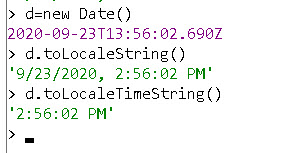### Converting Formatted Time Back to a Unix Timestamp

Sometimes you will you have data were the date and time is already formatted and you need to convert it back into a Unix type timestamp.

Here we use this from of the new date constructor

new Date(year, month, day, hours, minutes, seconds, milliseconds)

So if our date looks like this:

`2020-08-8,14:30:00`

Then we can use the following code to get a Unix Type time stamp:

```var Data="2020-08-8,14:30:00";
var data=Data.split(",");
var time=data;
data=data.split("-");

var year=data;
var month=data-1;    //notice the -1
var day=data

time=time.split(":");
var hours=time;
var mins=time;
var secs=time;
var d= new Date(year,month,day,hours,mins,secs);
msg.payload=d
msg.timestamp=d.getTime();
return msg;
```

If I paste the code into a function node and use the debug node to view the output this is what we see.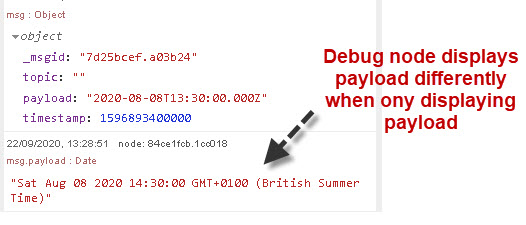You should also notice that one displays the local time and the other displays the time in GMT (UTC).

### Changing a Date

The data object has both get methods and set methods.

So if we wanted to change the hours we use:

``date.setHours(newValue)``

Example: Add 1 hour to current time

```var date=new Date();
var hour =date.getHours();
hour=hour+1;
date.setHours(hour);```

Note: in the above we would also need to check that we didn’t go past midnight in which case we would go on to the next day and would need code to take that into account.

Example: Add 1 hour to current time

```var date=new Date();
var timestamp =date.getTime();
timestamp=timestamp+(3600*1000); //1hour in milliseconds
```

Note: Now we don’t need to worry about the overflow into a new day which is why I find it easier to use a timestamp internally in my flows.

### Timezones

Universal Time (UTC) and Greenwich Mean Time (GMT) are the same thing, and time is store in the Date object in UTC.

When creating a date object the local time is assumed but you can pass in a Timezone offset to store the local time of another location in the date object.

There are two formats to use, You can use the time offset from GMT or the time zone abbreviation (PDT =Paciffic daylight time):

```new Date('September 26, 2018 19:20:00 PDT')
new Date('September 26, 2020 19:20:00 -0700')```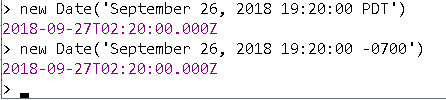A useful function for working with time zones is the getTimezoneOffset() which returns the minutes. Note in the screenshot below the local time is British summer time which is UTC+1 the offset returned is -60.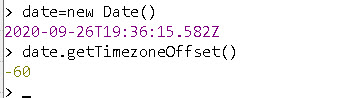If we had TTC+5 then -300 would be returned.

A list of offsets and abbreviations is here

### Summary

JavaScript as a whole collection of functions for working with time. Generally you store time a Unix type time stamp in Milliseconds and only convert it when it is displayed.

Likewise if you are working with different time zones then use Unix type time stamp internally and convert it for display.

Related Tutorials and Resources:

Click to rate this post!
[Total: 2 Average: 5]